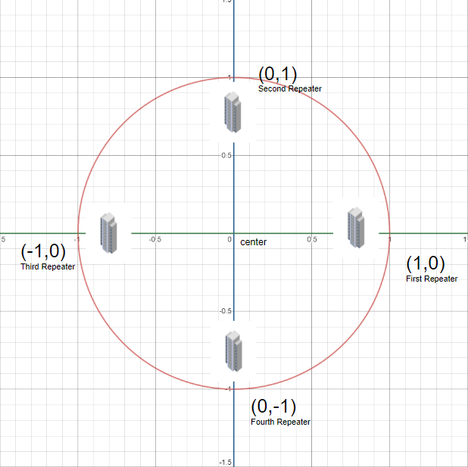# Placing the RepeaterpranonraianNational High School Prog...
Limits 1s, 512 MB

You are the mayor of a city named "HoZoBoRoLo". As the name suggests, the city is pretty weird. But the people are kind-hearted. Let's consider a brief map of the city. In Cartesian coordinate system, the town hall is in the center of the city and other buildings are within a fixed distance from the town hall. So we can think the city perimeter as a circle.

Like other cities, your city have also been affected by COVID-19 and you have been compelled to shut down all the educational institutions. As the students cannot lag behind, you want to start online classes. Currently, a major problem is: all the students don't have access to the internet at home. So you want to provide a free broadband internet connection to each of the students.

You asked your network department expert about the infrastructure. He told you that it would be best if a backbone connection is created throughout the perimeter of the city (shown in the picture using the red line). He added that as the perimeter is very large, you will have to put some repeater on the network line. He also suggested that, all repeaters should be placed as far as possible from the nearby repeaters.

Sadly, the city network department currently have $k$ repeaters in stock and due to pandemic, you cannot import repeaters from other cities.

Given the coordinates of the city town hall $(X_t, Y_t)$, the coordinates of the first repeater $(X, Y)$ and the number of repeaters in stock $k$, your task is to find the coordinates of the other repeaters so that each repeater is at the maximum distance from the nearby repeaters.

Use $pi = cos^{-1}(-1.0)$ if required.

## Input

First line of the input will contain a single integer $T$ denoting the number of testcases.

Next $T$ lines of the input contains five integers. $X_t, Y_t, X, Y, k$ where $(X_t, Y_t)$ denotes the coordinate of the townhall and $(X, Y)$ denotes the coordinate of the first repeater.

### Constraints

$1 \leq T \leq 25$
$-500 \leq X_t, Y_t ≤ 500$
$-500 \leq X, Y \leq 500$

$K = 2$

$2 < K \leq 180$

## Output

For each testcase, print $k - 1$ lines. Each line will have two numbers $(x, y)$ denoting the position of each repeater in a counter clockwise order from 1st repeater.

Absolute error less than $10^{-6}$ will be ignored.

## Samples

InputOutput
1
0 0 1 0 4

0 1
-1 0
0 -1


The image illustrates the position of each repeater.InputOutput
2
1 0 2 0 2
1 1 2 2 2

0 0
0 0


### Submit